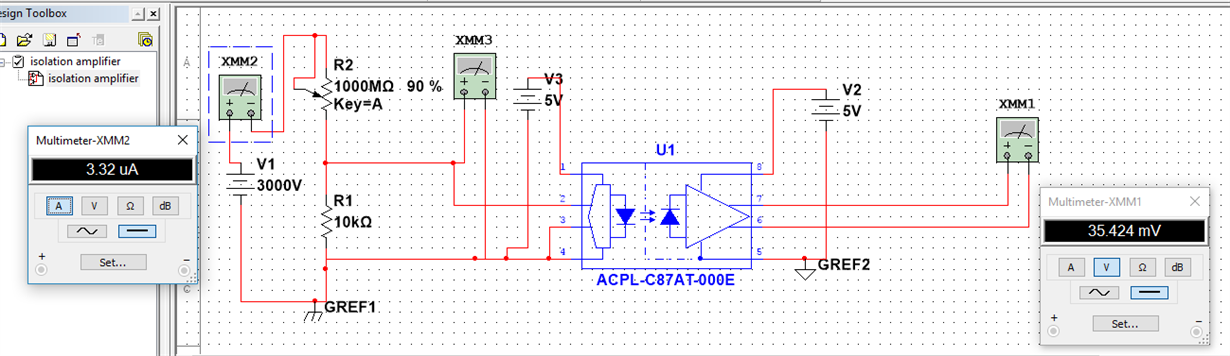If you have a related question, please click the "Ask a related question" button in the top right corner. The newly created question will be automatically linked to this question.

AMC1200EVM: High voltage current measurement

Part Number: AMC1200EVM
Other Parts Discussed in Thread: AMC1200, TIPD104

Hi, I want to measure a current from a high voltage isolated power supply (3000 volts, with ground is different (isolated) from circuit ground), The current measurement ranges from 1 microamps to 1000 microamps with a resolution of 1 microamps. I would like to measure the current either from low side or by measuring the differential voltage across a shunt resistor (1k ohm). Please let me know if i can use AMC1200EVM board to measure current or differential voltage (1 milivolts to 1 volts ). If not, please let me know the solution for measuring the current from isolated high voltage supply.

Regards.

Ismail

• Hi Ismail,

Welcome to our e2e forum! Can you share the schematic you intend to use with the AMC1200? The input voltage range to the AMC1200 is only +/-250mV, so your 1k shunt sounds a little high. Is the 3000V supply AC or DC?
• Dear Tom,

Thanks for your prompt reply, I do not have the exact schematic of AMC1200 for my circuit. Instead I have attached another schematic in which i need to replace ACPL-c87 with AMC 1200. Can I use a 100 ohm shunt resistor, so that the voltage across the resistor will be 0.1mV to 100 mV.  How can I find the resolution of AMC1200.• Hi Ismail,

The ACPL device has a 0-2V input range with a gain of 1, while the AMC1200 has a +/- .250V input range with a gain of 8. If you want to measure 1A at 100mV, you would need to use a 0.1 ohm shunt. Are you trying to measure current or voltage?
• Dear Tom,

My ultimate aim is to measure current for the high voltage power supply.

I am looking for the solution similar to this one. (Isolated Current Sensing Reference Design Solution, 5A, 2kV) The difference is my current ranges from (1 micro amp to 1 milli amp). Towards this requirement, if I calculate the value of shunt resistance Rsh(max) = 250 mV / 1 mA = 250 ohm. So that I will get Vout = 2V.  However when my minimum current is 1 microamps. The shunt resistance voltage is 250 micro Volts, and the Vout = 8*250 micro volts = 2 milli volts.

My question is: can I able to observe the value of 2 mili volts at the output?

Or Can I go for option 2:

Current in the range of 1 micro amps to 200 micro amps:

Rsh(max) = (250mV / 200 microamps) = 1.25 k ohm

minimum Vin= 1.25 k ohm * 1 microamps = 1.25 mili volts.

minimum Vout = 8 * Vin =  8 * 1.25 milli Volts = 10 milli Volts.

www.ti.com/.../slau521.pdf

• Hi Ismail,

probably a logarithmic amplifier will do a good Job here ..

With best regards

Gerhard

• Dear Tom,